# Measurement of sound

#### asdasd12e12

Joined Nov 24, 2021
22
I'm trying to develop what is shown in the image below :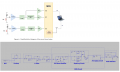A sound level meter where through its input i can show the rms value in a computer screen. For now i'm just trying to do the analog front end. The LTspice part is what i have.

Starting with the MIC and pre amplifier, i used as an input the sound of some animal farms and amplified that 5 times so the next stages of the circuit could work better with the signal. If i plan to do this in a real circuit board i need to bias the FET that is inside the MIC with a resistor and DC couple the input signal with a capacitor, i didnt do that here because there's no transistor, i simulated the MIC with just a voltage source. I'm not sure if with an actual circuit board things would go differently.

Here is the result: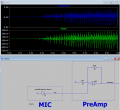Next the signal goes through an A weighting filter (trys to emulate the perceived human ear in function of frequency):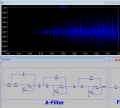With this input signal at the Mic, there's an attenuation because the amplitude of the output signal in the A-filter is lower than the amplitude of the signal that entered the A-filter.

I would say this is due to the frequency of the input signal being in the zone where the A-filter makes the signal go lower (the figure in the right is the frequency response of the A-filter):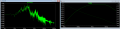If i didnt amplify the signal in the beggining i would have almost a 0V signal at the end.

Next stages are the full wave rectifer and the squaring circuit. So to compute the rms i need to first square the signal than compute an average out of that, one problem that i had was that the squaring circuit (= multiplier circuit) isnt valid for the third quadrant, if the input is negative it wont work.
So there was need to make a full wave rectifier to convert the negative values of the input to positive: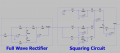And the results: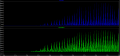Everything here goes well except there are some instances where the values for Vmulti (after multiplying the input with itself) go negative, i think the multiplier circuit is not very sensitive to low voltage values but is this best i have right now.

After that i need to do an average and i made that according to this: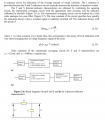Where i have can have 3 RC circuits with different time constants. One of them require the peak value to be measured, it just makes reading the measurement easy as it has a short time constant. The one made has a time constant of 35ms.

Here is a better view of the circuit: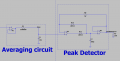And here is the result: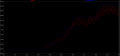I still need to make a square root of the output but i could do that in the MCU. The next stage would be an amplifier to scale the output signal in a way that i would make better use of the MCU resolution, it isnt done yet because i still didnt chose a MCU.

One of the questions that i have is how will i convert the rms value to SPL according to this: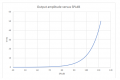I have to keep in mind that i amplified the signal one time in the beginning where the feedback resistor can always change (if the signal has a very low amplitude i need to amplify more) and then one more time in the end of the AFE. So the rms value in the input may not be the 'real' one, what should i do?

There's concerns about the calibration, i have no idea how to make it here. All i saw about this is that the preAmp stage is what i should use for calibration, where i use as an input some wave where i know its SPL and keep changing the feedback resistor till in the MCU i get the same SPL value but that doesnt seem very pratical in my case, the biggest reason being that i have no ways of garanting that i can emit a SPL known input.
I tested this with a sine wave as a mic input without the pre amplifier and i got the a very close value for the rms

I also would like to hear more opinions about the things i did hear aswell if possible. thanks.

#### Audioguru again

Joined Oct 21, 2019
4,945
An A-weighing filter in a sound level meter is used only when the sound levels are very low. There is almost no filtering for high audio levels. Medium sound levels need only a little filtering.
Two colors on the display can show average and peak levels at the same time.

#### MrAl

Joined Jun 17, 2014
9,349
I'm trying to develop what is shown in the image below :

View attachment 273298

A sound level meter where through its input i can show the rms value in a computer screen. For now i'm just trying to do the analog front end. The LTspice part is what i have.

Starting with the MIC and pre amplifier, i used as an input the sound of some animal farms and amplified that 5 times so the next stages of the circuit could work better with the signal. If i plan to do this in a real circuit board i need to bias the FET that is inside the MIC with a resistor and DC couple the input signal with a capacitor, i didnt do that here because there's no transistor, i simulated the MIC with just a voltage source. I'm not sure if with an actual circuit board things would go differently.

Here is the result:

View attachment 273290

Next the signal goes through an A weighting filter (trys to emulate the perceived human ear in function of frequency):

View attachment 273291

With this input signal at the Mic, there's an attenuation because the amplitude of the output signal in the A-filter is lower than the amplitude of the signal that entered the A-filter.

I would say this is due to the frequency of the input signal being in the zone where the A-filter makes the signal go lower (the figure in the right is the frequency response of the A-filter):

View attachment 273292

If i didnt amplify the signal in the beggining i would have almost a 0V signal at the end.

Next stages are the full wave rectifer and the squaring circuit. So to compute the rms i need to first square the signal than compute an average out of that, one problem that i had was that the squaring circuit (= multiplier circuit) isnt valid for the third quadrant, if the input is negative it wont work.
So there was need to make a full wave rectifier to convert the negative values of the input to positive:

View attachment 273293

And the results:

View attachment 273294

Everything here goes well except there are some instances where the values for Vmulti (after multiplying the input with itself) go negative, i think the multiplier circuit is not very sensitive to low voltage values but is this best i have right now.

After that i need to do an average and i made that according to this:

View attachment 273295

Where i have can have 3 RC circuits with different time constants. One of them require the peak value to be measured, it just makes reading the measurement easy as it has a short time constant. The one made has a time constant of 35ms.

Here is a better view of the circuit:

View attachment 273296

And here is the result:

View attachment 273297

I still need to make a square root of the output but i could do that in the MCU. The next stage would be an amplifier to scale the output signal in a way that i would make better use of the MCU resolution, it isnt done yet because i still didnt chose a MCU.

One of the questions that i have is how will i convert the rms value to SPL according to this:

View attachment 273299

I have to keep in mind that i amplified the signal one time in the beginning where the feedback resistor can always change (if the signal has a very low amplitude i need to amplify more) and then one more time in the end of the AFE. So the rms value in the input may not be the 'real' one, what should i do?

There's concerns about the calibration, i have no idea how to make it here. All i saw about this is that the preAmp stage is what i should use for calibration, where i use as an input some wave where i know its SPL and keep changing the feedback resistor till in the MCU i get the same SPL value but that doesnt seem very pratical in my case, the biggest reason being that i have no ways of garanting that i can emit a SPL known input.
I tested this with a sine wave as a mic input without the pre amplifier and i got the a very close value for the rms

I also would like to hear more opinions about the things i did hear aswell if possible. thanks.
Hello,

In converting the RMS to the required response you should probably program that into the MCU you choose. That's because it will probably be difficult to do that in pure analog where you need repeatability as well as the right shape function. You could check the curve and see if it matches that of a diode, or use a diode network to shape the output (along with an amplifier most likely) but that technique tends to be temperature sensitive. Using the programmed MCU you could provide a table and interpret between table points with either a simple two point interpretation technique or a more complex one that takes into account three points and some derivatives (think cubic spline) or more points. I would think that would be accurate enough.

#### MrChips

Joined Oct 2, 2009
26,792
I would have taken a different approach, one that is more software intensive.
I would have amplified the signal from the microphone first. The next step would be to apply an anti-aliasing filter and then digitize it on the MCU. After that, all further processing can be accomplished in software.

•MarcosKDC, MrAl and LowQCab

#### LowQCab

Joined Nov 6, 2012
2,342
I'm an Analog kinda guy, but your MCU can do this very well with the right programming.
What I'm wondering is, what's the point of having this Computer-Display ?,
what are You trying to achieve with this project ?
Why are there 2-Channels ?,
and why are You bypassing some of your proposed processing on one Channel ?

SPL-Meters are fairly cheap, and work well.
.
.
.

#### MrAl

Joined Jun 17, 2014
9,349
I would have taken a different approach, one that is more software intensive.
I would have amplified the signal from the microphone first. The next step would be to apply an anti-aliasing filter and then digitize it on the MCU. After that, all further processing can be accomplished in software.
Hi,

I was assuming that he wanted to work in analog as much as he could, but you are right if you are using a MCU then why would you want to use analog with that if you didnt really have to.
So many things can be done these days with simple and low cost MCU's i wonder if anything is analog anymore#### MrChips

Joined Oct 2, 2009
26,792
One of my research endeavors was to eliminate the analog front end as much as possible.
We would digitize the data right at the sensor thus reduce the chances of the data being corrupted with external interference in the analog circuitry. We used 24-bit ADC with better than 1μV resolution. This pushed the focus on to sensor design in any data acquisition system.

•MarcosKDC